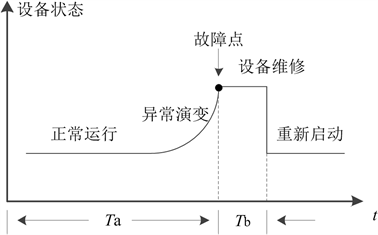# 基于全寿命周期成本模型的输变电设备检修策略研究Study on the Maintenance Strategies of Transmission and Transformation Equipment Based on Life Cycle Cost Model

• 全文下载: PDF(497KB)    PP.158-162   DOI: 10.12677/AEPE.2017.56024
• 下载量: 517  浏览量: 1,466

The factor of LCC (Life Cycle Cost) is not considered in the present process of making maintenance strategy for power equipment, which leads to the failure of the maintenance strategy to maximize the economic benefits. In this paper, these common maintenance strategies for transmission and transformation equipment are corrected based on the LCC model considering time based mainte-nances and condition based maintenance. On the premise of ensuring the safe and stable operation of the system, some reasonable maintenance strategies of highly valuable in engineering are formulated, and the economic goal is realized.

1. 引言

2. 电力设备全寿命周期成本模型

$LCC={C}_{I}+{C}_{O}+{C}_{M}+{C}_{\mathrm{F}}+{C}_{D}$ (1)

2.1. 初始投资成本CI

${C}_{\text{I}}=\sum {C}_{\text{I}n}\text{\hspace{0.17em}}\left(n=1,2,3,\cdots \right)$ (2)

2.2. 运行成本CO

2.3. 维护成本CM

$CM=\frac{T}{{T}_{\text{a}}+{T}_{\text{b}}}×\underset{j=1}{\overset{q}{\sum }}C{M}_{j}×{f}_{j}$ (3)

2.4. 故障成本CF

${C}_{F}=\lambda {C}_{R}+{W}_{\mathrm{EENS}}\epsilon +{W}_{EENS}r$ (4)

2.5. 退役处置成本CDFigure 1. State transition diagram on the equipment that can repair

${C}_{D}=-{C}_{I}×\alpha \text{%}$ (5)

2.6. 对LCC模型进行经济学修正

${C}_{p}=\underset{t=1}{\overset{n}{\sum }}{C}_{t}×\frac{{\left(1+R\right)}^{t}}{{\left(1+r\right)}^{t}}$ (6)

3. 电力设备检修策略

1) 事故后维修是指当电力设备发生故障或事故时进行的维修，这种检修制度以设备是否损坏为依据，属于被动式维修。

2) 定期检修(Time Based Maintenance, TBM)是指按照检修规程、以固定时间为基础的检修方式，属于预防性检修。但是由于其忽略了不同设备的现状(磨损、使用情况等)，可能导致维修过度或不足，对设备本身造成一定损伤。

3) 状态检修(Condition Based Maintenance, CBM)是指基于设备当前的状态，通过各种状态监测技术，识别故障的早期征兆，对故障部位、严重程度及发展趋势做出预判，从而确定各设备的最佳维修时间  。随着在线监测技术和故障诊断技术的不断发展，状态检修逐渐走向实用化，与定期检修相比，状态检修主要有以下几点  ：

1) 状态检修可根据输变电设备的运行现状，通过综合分析来确定设备是否需要进行检修，何时进行检修，因此针对性更强。

2) 对于状态较好的设备，状态检修能延长维修周期，节省大量的人力、物力，避免不必要的浪费。

3) 对于状态较差的设备，状态检修能缩短检修周期，提高供电可靠性。

4. 基于LCC模型的不同检修策略修正

4.1. 定期检修(TBM)策略

$C{M}_{\text{TBM}}=\frac{T}{{T}_{\text{a}}{}_{\text{TBM}}+{T}_{\text{bTBM}}}×\underset{j=1}{\overset{q}{\sum }}C{M}_{j}×{f}_{j}$ (7)

4.2. 状态检修(CBM)策略

1) 初始投资成本

2) 维护成本

5. 结论

1) 当前LCC模型中存在一些不足，如未考虑不同检修策略下模型的不同，同时经济学修正时应同时考虑折现率和通货膨胀率等。

2) 目前电力系统的检修策略主要为定期检修和状态维修相结合的方式。

3) 针对不同检修策略，建立其相应的LCC模型，能够在保证系统安全稳定运行的前提下，制定出合理的检修策略，实现经济性的目标。

  郭丽杰. 基于风险的石化动设备智能维修决策研究[D]: [博士学位论文]. 北京: 化工大学, 2009.  王丹, 邢丽敏. 变电站设备状态检修技术及其应用探讨[J]. 科技创新导报, 2011(30): 76.  Nilsson, J. and Bertling, L. (2007) Maintenance Management of Wind Power Systems Using Condition Monitoring Systems-Life Cycle Cost Analysis for Two Case Studies. IEEE Transactions on Energy Conversion, 22, 223-229.  滕乐天, 陈红兵, 王怡风. LCC在设备采购中的应用实践[J]. 华东电力, 2007, 35(10): 27-29.  苏海锋, 张建华, 梁志瑞, 等. 基于LCC管理的高压直流输电线路工程设计方案评价研究[J]. 华东电力, 2011(5): 698-701.  郭基伟, 柳纲, 唐国庆, 王英. 电力设备检修策略的马尔可夫决策[J]. 电力系统及其自动化学报, 2004(16): 6-10.  张大波. 基于状态监测与系统风险评估的电力设备维修及更新策略研究[D]: [博士学位论文]. 重庆: 重庆大学, 2012.  许婧, 王晶, 高峰, 等. 电力设备状态检修技术研究综述[J]. 电网技术, 2000, 24(8): 48-52.  宋秀省. 电力设备状态检修技术研究[J]. 低碳世界, 2013(16): 66-67.  李刚, 于长海, 刘云鹏, 等. 电力变压器故障预测与健康管理: 挑战与展望[J]. 电力系统自动化, 2017: 1-12.  冀凯琳. 基于LCC理论的电力设备检修策略研究[D]: [硕士学位论文]. 北京: 华北电力大学(北京), 2011.